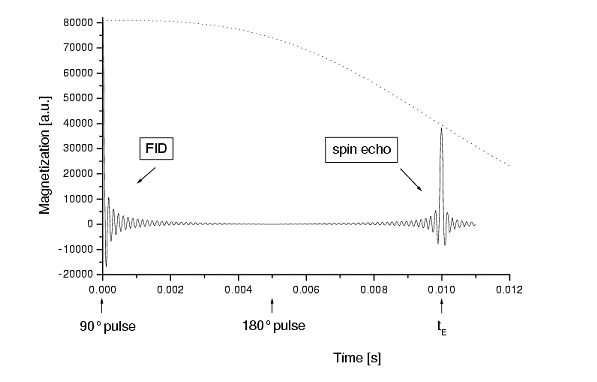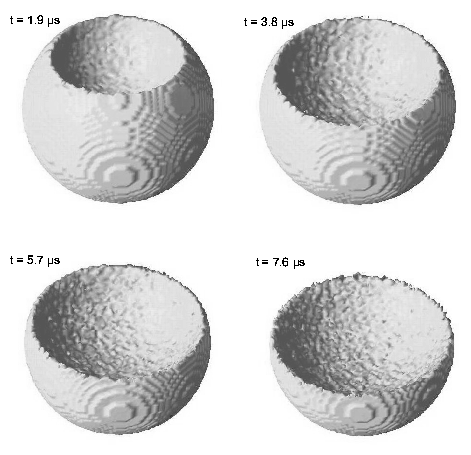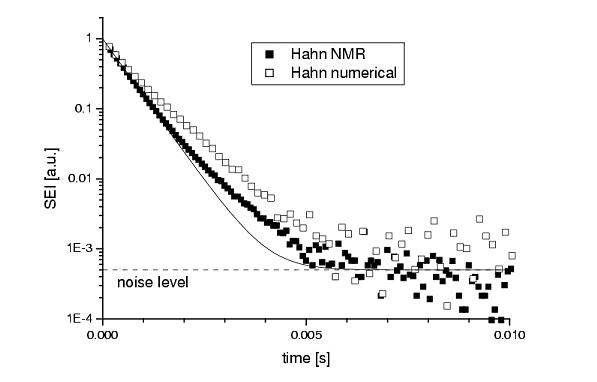Iron influence on Relaxometry

The interpretation of the relaxometry results in terms of a pore-size distribution for building materials leads to research on the so-called NMR dephasing behavior. This NMR effect is absent or not important in most porous materials. However, building materials contain high amounts of iron oxide, which will give additional magnetic fields. Therefore the NMR dephasing behavior in these high internal magnetic fields is of importance for the relaxometry measurements. A short description of the results is given below. Also references are included for more detailed texts about this topic.

NMR Hahn and CPMG spin-echo measurements showed such a complex spin-echo decay, that it was decided to develop a numerical model. In this model, the magnetic moments of nuclei accumulate a certain phase, depending on their random trajectory through an in-homogeneous magnetic field. The results of this numerical model for classical situations (e.g., free diffusion in a uniform magnetic-field gradient) are in perfect agreement with the well-known analytical solutions. Figure 1., e.g., shows the simulated magnetization as a function of time for a Hahn spin-echo.Figure 1: The simulated magnetization as a function of time for a Hahn spin-echo.

We continued with simulations of the dephasing behavior of nuclear spins in a spherical pore. First, a uniform gradient was assumed in this pore. Using these simulations the existing theory, which predicts two asymptotical dephasing regimes (`motional averaging and `localization'), has been verified and extended with an `intermediate regime'. Next, in the model a dipolar magnetic field was created inside the pore, by putting a magnetic point-dipole in the solid matrix surrounding the pore. The decay of the simulated spin-echoes can be predicted with scaling laws. For the `motional averaging regime' with a homogeneously distributed nuclear magnetization in the pore, this relation could be derived directly from existing theories. However, for the `localization regime' with an inhomogeneously distributed magnetization, these scaling laws were derived from basic principles by ourselves.Figure 2: The iso-magnetization surface of half the equilibrium magnetization for four succesive spin-echo times.
The magnetic dipole (strength=10E-14 Am^2) is situated at the top of the pore with a radius of 3 µm)

In figure 2 all spins with a magnetization of half the equilibrium magnetization is shown for four successive spin-echo times. In this simulation, the magnetic dipole is situated at the top of the pore. For a detailed description of the magnetization decay and the explanation of figure 2, the reader is reffered to J.Chem. Phys. 2001.Figure 3: Hahn spin-echo intensities as a function of the spin-echo time for a 4.3% weight Fe2O3 doped sample. The solid squares denote experimental data. The open squares reflect the corresponding model prediction. The solid curve represents a fit to the experimental data of a sum of a mono-exponential decay and an appropriate noise level (dashed line).

The NMR spin-echo measurements on a series of magnetically doped clay samples were done. In this paper, the dephasing effects are explained without the use of the model. However, further analysis of Hahn and CPMG spin-echo measurements with a much better signal-to-noise ratio revealed behavior that can only be explained with the random-walk model. The measured spin-echo decay agrees with the results of the model of a dipolar magnetic field inside a pore. Therefore, in the model, the magnetic impurities are described as additional magnetic point-dipoles with a strength depending on the doping fraction. The experimental results agree well with the predictions from this model, as can be seen in figure 3 for a Hahn spin-echo measurement. This holds also for the CPMG measurements, up till spin-echo times for which the diffusion length becomes comparable to the pore size.

• An extensive description of this model can be found in:
• R.M.E. Valckenborg, H.P. Huinink, J.J. v.d. Sande, and K. Kopinga, Random walk simulations of NMR dephasing effects due to uniform magnetic field gradients in a pore, Phys. Rev. E. 65, 021306 (2002)

R.M.E. Valckenborg, L. Pel, and K. Kopinga, NMR relaxation and diffusion measurements on iron(III) doped Kaolin clay, J. Mag. Res. 151, 291-297 (2001).

R.M.E. Valckenborg, NMR on technological porous materials, Ph.D. thesis, Eindhoven University of Technology (2001).

R.M.E. Valckenborg, H.P. Huinink and K. Kopinga; NMR Dephasing Effects due to a magnetic dipole in a spherical pore, Journal of Chemical Physics 118, 3243-3251 (2003)